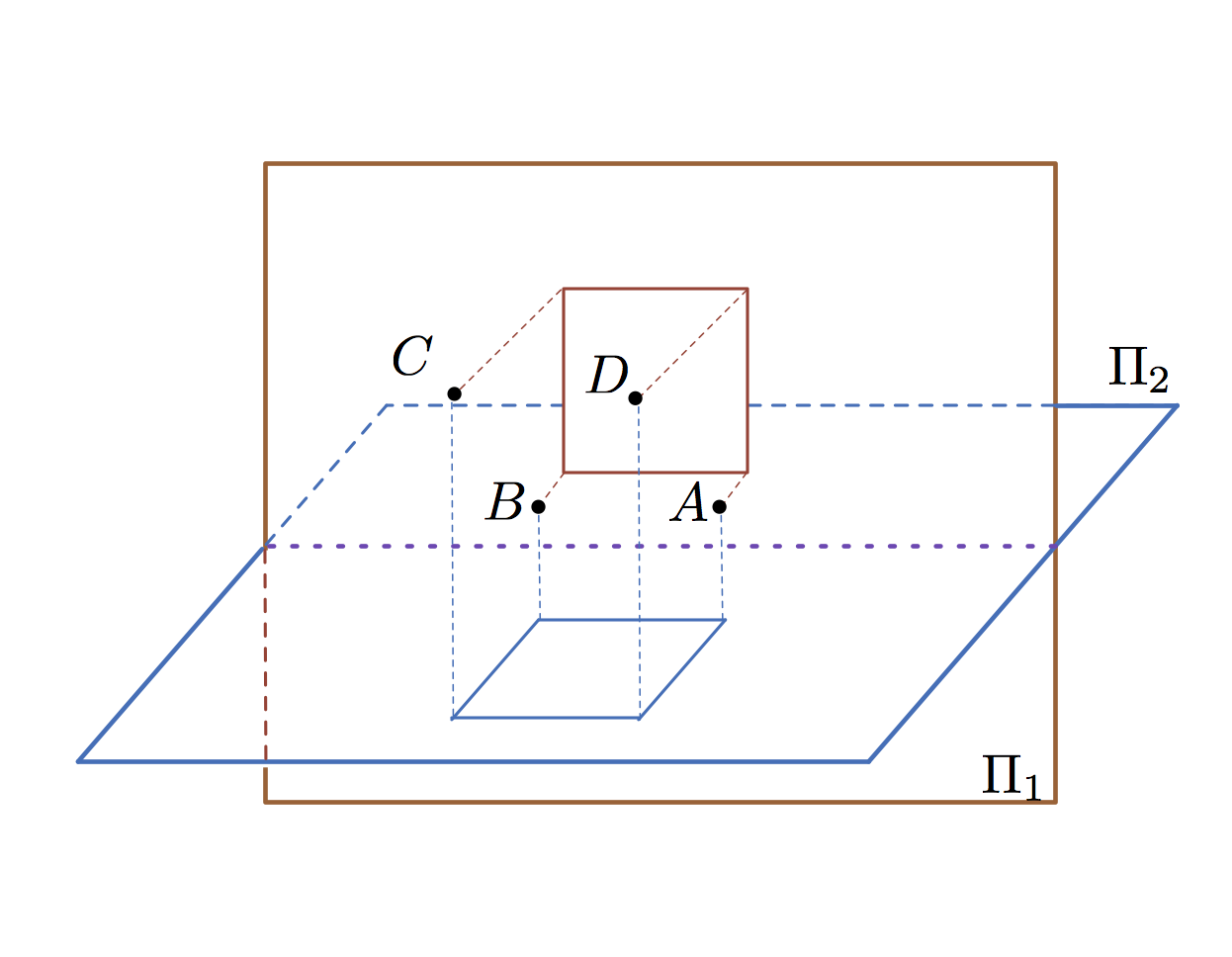# Almost A Square, But Not QuiteSuppose $A, B, C$ and $D$ are 4 points in space that lie on the same plane, with $ABCD$ being a quadrilateral. There are 2 perpendicular planes $\Pi_1$ and $\Pi_2$, such that the orthogonal projections of $ABCD$ onto these planes form a square of area $123$ each. If $|AB|=12,$ what is the perimeter of $ABCD$ to 1 decimal place?

×